Solving Equations

The graphing calculator can be easily used to solve and/or check algebraic equations.

 Example 1:    Solve for x:   4x + 1 = 9 Enter the left side of the equation into Y1. Enter the right side of the equation into Y2. Graph  (you may need to adjust your window to see where the two graphs intersect) Find the point of intersection (the answer). (2nd CALC (above TRACE), #5 Intersect) Answer:   x = 2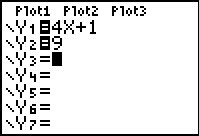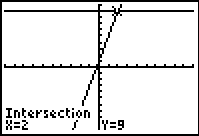Example 2:    Solve for x:   2x + 4 = 5x - 2 Answer:   x = 2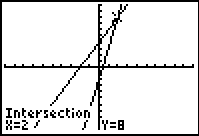Example 3:    Solve for x:   x2 + x = x2 - 2x + 6 Answer:  x = 2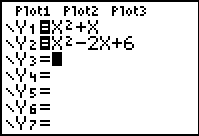Example 4:.   Solve for x:   15x - 3(3x + 4) = 6.  Choose the correct value for x. 1) 1       (2)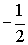(3) 3       (4)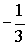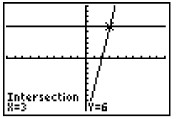Finding Your Way Around TABLE of  CONTENTS